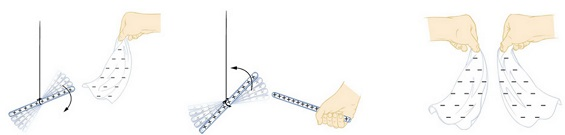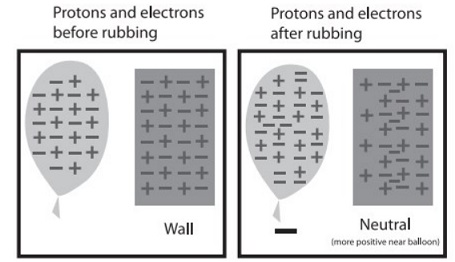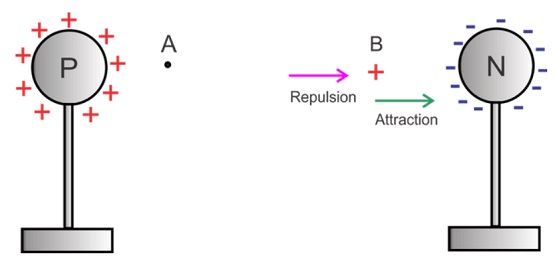# What is Electric Charge

## ELECTRIC CHARGE

Electric charge, also called "the Quantity of Electricity," is a fundamental component of everyday matter. Objects are made of molecules and atoms, atoms are made of protons, neutrons, and electrons, and the protons and electrons are made in part out of electric charge. Electric charge is substance-like. If you have a quantity of charge, you cannot destroy it, you can only move it from place to place. A flow of electric charge is called "electric current.

Q = l.t

From the study of atomic structure we know that an atom consists of a central part called nucleus and around the nucleus (called extra-nucleus) there are a number of electrons revolving in different paths or orbits. The nucleus consist of protons and neutrons. A proton is a positively charged particle while a neutron has no charge. Therefore, the nucleus of an atom bears a positive charge. An electron is a negatively charged particle having magnitude of negative charge equal to the magnitude of positive charge on a proton. Normally, the number of electrons is equal-to-the number of protons in an atom. Therefore, an atom is neutral as a whole, the negative charge on electrons cancelling the positive charge on protons. This leads to the conclusion that under ordinary conditions, a body is neutral, i.e. it exhibits no charge. When a body has deficiency or excess of electrons from the normal, it is said to be charged or electric field.

### TYPES OF CHARGE :

There are two types of charges known as positive and negative charges. All objects normally contain equal amount of positive and negative charges and are therefore, electrically neutral.

If an object has the same amount of positive and negative charge the NET CHARGE on the object is the sum of the two kinds of charge and adds to zero, and we say the object is electrically neutral. So if an object has a number of protons equal to the number of electrons its net charge is zero. (All atoms have a net charge of zero, but ions can have a positive or negative charge depending on whether they have more protons or electrons.) Since the electrons are more mobile than the protons (which are buried in the nucleus of the atom) we transfer electrons to a neutral object to make it have a net negative charge. When we do that the neutral object from which we transferred the electrons becomes positively charged. Note that the total electric charge of the two bodies together does not change. This is known as the conservation of charge. We cannot create or destroy electric charge, we simply transfer charges (electrons) from one object to another to change the NET charge of objects.

The ancient Greeks discovered that electric charge could be transferred between two objects by rubbing them together. In the diagram below, rubbing plastic and fur together results in electrons from the fur being rubbed off onto the plastic, leaving the fur positively charged and the plastic negatively charged. When glass and silk are rubbed together which one has the electrons rubbed off?Plastic rubbed with fur becomes negatively charged,  glass rubbed with silk becomes positively charged.

When we comb dry hair, the comb gets charged and can pick small pieces of paper brought near it. When we rub a glass rod with silk cloth or a piece of ebonite rod with woolen material, the charge acquired by a glass rod rubbed with silk is called a positive charge and that on ebonite rod, is called a negative charge.

Although the charges (on the electrons) are tightly bound to the atoms in an insulator they are free to move slightly within the atom. This is called polarization. If a plastic comb is rubbed on fur (or your dry hair) electrons will be rubbed off the hair onto the plastic comb. The plastic comb becomes charged negatively. If the comb is brought close to a neutral insulator, like a piece of dry paper, it will repel the negatively charged electrons in the atoms causing them to moving away slightly, leaving the protons without an electron closer to the comb. Since opposite charges attract and the positive charges are closer to the comb than the negative charges, the piece of paper is attracted to the comb. This effect is used to remove soot and ashes from smoke going up industrial chimneys. The inventor became rich as a result of his patent.(a)

A charged comb attracts a piece of paper.

Part (b) of the diagram shows the same result (attraction of the paper) even if we had a positively charged comb.

Glass rod and ebonite rod will attract each other while two glass rods as well as two ebonite rods will repel each other.

### PROPERTIES OF CHARGE:

(i) Like charges repel each other and unlike charges attract each other.

(ii) The charge is quantized i.e. charge on an object is equal to ± ne where n is an integer and

e = 1.6 × 10-19 C.

(iii) Electric charge is a scalar quantity.

(iv) The electric charge on a system is always conserved.

### UNIT OF CHARGE :

The charge on an electron is so small that it is not convenient to select it as the unit of charge. In practice, coulomb is used as the unit of charge, i.e. SI unit of charge is coulomb abbreviated as C. One coulomb of charge is equal to the charge on 6.25 × 1016 electrons.

1 coulomb = charge on 6.25 × 1018 electrons

Thus, when we say that a body has a positive charge of one coulomb (i.e. + 1 C) it means that the body has a deficit of 6.25 × 1018 electrons from the normal due share.

The charge on one electron in coulomb is given by:

– e = –1.6 × 10-19 C.

1.6 × 10-19 C.

### FLOW OF CHARGE:

Consider two metallic spheres A and B which are placed on the insulated stands as shown in the figure. Let sphere A is positively charged and sphere B is negatively charged. Let these spheres are connected by a metallic wire. The electrons flow from sphere B (at lower potential) to the sphere A (at higher potential). The flow of electrons continues till the potential of both the spheres becomes equal. This shows that the flow of charge (i.e. electrons) between two points continues as long as they are at different potentials or as long as there is potential difference between these two points.